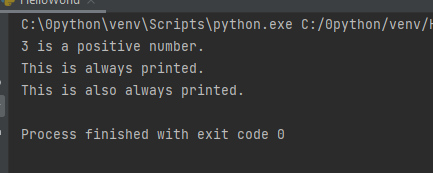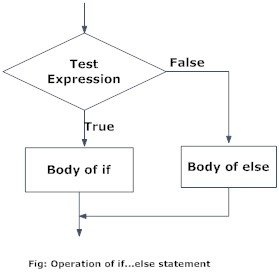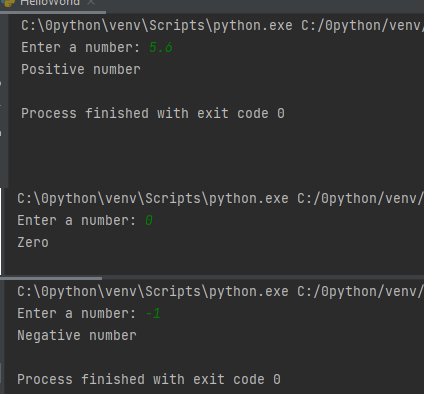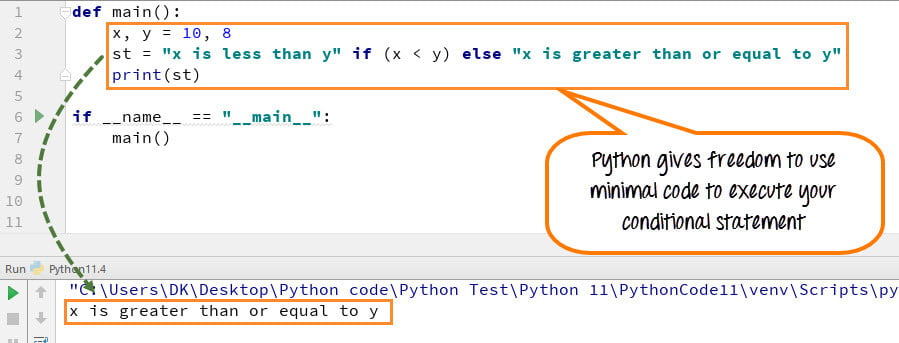# Python if…else语句 （条件语句）

## 1.Python 中的`if...else`语句是什么？

`if…elif…else`语句在 Python 中用于决策。

### Python `if`语句语法

```if test expression:
statement(s)
```

Python 将非零值解释为True。None和0解释为False。

```# If the number is positive, we print an appropriate message

num = 3
if num > 0:
print(num, "is a positive number.")
print("This is always printed.")

num = -1
if num > 0:
print(num, "is a positive number.")
print("This is also always printed.")
```print()语句位于if块之外（未缩进）。 因此，无论测试表达式如何，都将执行它。

## 2.Python `if...else`语句

### `if...else`的语法

```if test expression:
Body of if
else:
Body of else
```

if..else语句求值test expression并仅在测试条件为True时才执行if的主体。```# Program checks if the number is positive or negative
# And displays an appropriate message

num = 3

# Try these two variations as well.
# num = -5
# num = 0

if num >= 0:
print("Positive or Zero")
else:
print("Negative number")
```

``Positive or Zero``

## 3.Python `if...elif...else`语句

### `if...elif...else`的语法

```if test expression:
Body of if
elif test expression:
Body of elif
else:
Body of else
```

elif是if的缩写。 它允许我们检查多个表达式。

if块只能有一个else块。 但是它可以具有多个elif块。

if…elif…else的流程图

```'''In this program,
we check if the number is positive or
negative or zero and
display an appropriate message'''

num = 3.4

# Try these two variations as well:
# num = 0
# num = -4.5

if num > 0:
print("Positive number")
elif num == 0:
print("Zero")
else:
print("Negative number")
```

``Positive number``

```# Example file for working with conditional statement
#
def main():
x, y = 8, 8

if (x < y):
st = "x is less than y"

elif (x == y):
st = "x is same as y"

else:
st = "x is greater than y"
print(st)

if __name__ == "__main__":
main()

```

``x is same as y``
• 代码行 5：我们定义了两个变量 x, y = 8, 8。
• 代码第 7 行：if 语句检查条件 x 代码第 10 行：程序控制流程进入 elseif 条件。它检查 x==y 是否为真。
• 代码第 11 行：变量 st 设置为“x 与 y 相同”。
• 代码第 15 行：程序控制流退出 if 语句（它不会到达 else 语句）。并打印变量 st。输出是“x is same as y”，这是正确的。

## 4. Python 嵌套`if`语句

```'''In this program, we input a number
check if the number is positive or
negative or zero and display
an appropriate message
This time we use nested if statement'''

num = float(input("Enter a number: "))
if num >= 0:
if num == 0:
print("Zero")
else:
print("Positive number")
else:
print("Negative number")
``````
total = 100
#country = "US"
country = "AU"
if country == "US":
if total <= 50:
print("Shipping Cost is  \$50")
elif total <= 100:
print("Shipping Cost is \$25")
elif total <= 150:
print("Shipping Costs \$5")
else:
print("FREE")
if country == "AU":
if total <= 50:
print("Shipping Cost is  \$100")
else:
print("FREE")
```

``Shipping Cost is \$25``

## 5.如何用最少的代码执行条件语句

``A If B else C``

```def main():
x, y = 10, 8
st = "x is less than y" if (x < y) else "x is greater than or equal to y"
print(st)

if __name__ == "__main__":
main()
```

``x is greater than or equal to y``How to execute conditional statement with minimal code
• 代码行 2：我们定义了两个变量 x, y = 10, 8
• 代码行 3：变量 st 设置为“x 小于 y”，如果 x<y，否则设置为“x 大于或等于 y”。在这个 x>y 变量中，st 被设置为“x 大于或等于 y”。
• 代码行 4：打印 st 的值并给出正确的输出
• Python 无需为条件语句编写长代码，而是让您可以自由地以简洁明了的方式编写代码。

Posted in Python教程